Test: Data Handling - 2

# Test: Data Handling - 2

Test Description

## 10 Questions MCQ Test Mathematics for Class 3: NCERT | Test: Data Handling - 2

Test: Data Handling - 2 for Class 3 2022 is part of Mathematics for Class 3: NCERT preparation. The Test: Data Handling - 2 questions and answers have been prepared according to the Class 3 exam syllabus.The Test: Data Handling - 2 MCQs are made for Class 3 2022 Exam. Find important definitions, questions, notes, meanings, examples, exercises, MCQs and online tests for Test: Data Handling - 2 below.
Solutions of Test: Data Handling - 2 questions in English are available as part of our Mathematics for Class 3: NCERT for Class 3 & Test: Data Handling - 2 solutions in Hindi for Mathematics for Class 3: NCERT course. Download more important topics, notes, lectures and mock test series for Class 3 Exam by signing up for free. Attempt Test: Data Handling - 2 | 10 questions in 20 minutes | Mock test for Class 3 preparation | Free important questions MCQ to study Mathematics for Class 3: NCERT for Class 3 Exam | Download free PDF with solutions
 1 Crore+ students have signed up on EduRev. Have you?
Test: Data Handling - 2 - Question 1

###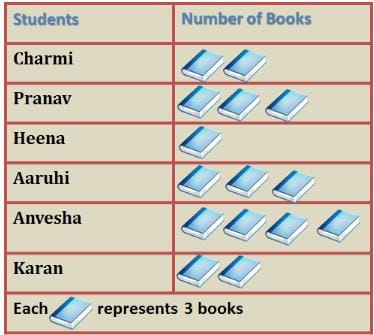How many more books did Anvesha read than Heena?

Detailed Solution for Test: Data Handling - 2 - Question 1

Number of books read by each = number of picture of book x number of books represented by each picture

Number of books read by Heena =

1 x 3 = 3 books

Number of books read by Anvesha =

4 x 3 = 12 books

Difference = 12 - 3 = 9 books

Test: Data Handling - 2 - Question 2

### DIRECTION: The given bar graph shows the amount of money spent by Amit in a week. Study the graph and answer the questions.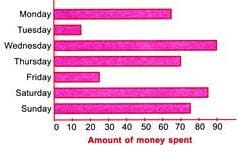Q. How much more amount Amit spent on Thursday than on Tuesday?

Detailed Solution for Test: Data Handling - 2 - Question 2

Amount spent on Thursday = Rs. 70 Amount spent on Tuesday = Rs. 15 So, more amount spent on Thursday than on Tuesday = Rs. 70 − Rs. 15 = Rs. 55

Test: Data Handling - 2 - Question 3

### DIRECTION: The given bar graph shows the amount of money spent by Amit in a week. Study the graph and answer the questions.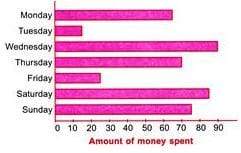Q. On which day Amit spent Rs. 65?

Detailed Solution for Test: Data Handling - 2 - Question 3

According to the bar graph, on Monday Amit spent Rs. 65.

Test: Data Handling - 2 - Question 4

DIRECTION: The given bar graph shows the amount of money spent by Amit in a week. Study the graph and answer the questions.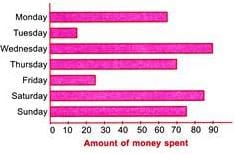Q. Find the amount of money spent by Amit on Sunday.

Detailed Solution for Test: Data Handling - 2 - Question 4

Amount of money spent by Amit on Sunday = Rs. 75.

Test: Data Handling - 2 - Question 5

DIRECTION: The given bar graph shows the amount of money spent by Amit in a week. Study the graph and answer the questions.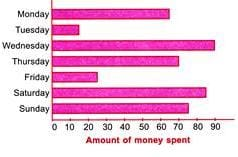Q. How much less Amount Amit spent on Friday than on Wednesday?

Detailed Solution for Test: Data Handling - 2 - Question 5

Amount spent on Friday = Rs. 25 Amount spent on Wednesday = Rs. 90
∴ Required difference = Rs. 90 − Rs. 25 = Rs. 65. So, Amit spent Rs. 65 less on Friday than on Wednesday.

Test: Data Handling - 2 - Question 6

DIRECTION: The given graph shows the number of people who attended a book fair. Study it carefully and answer the following questions.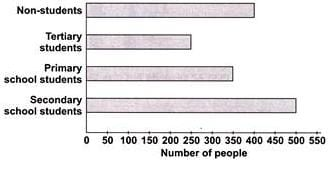Q. The number of secondary school students was twice the number of________.

Detailed Solution for Test: Data Handling - 2 - Question 6

Number of secondary school students = 500
Number of tertiary students = 250
∴ Number of secondary school students = 2 × number of tertiary students

Test: Data Handling - 2 - Question 7

DIRECTION: The given graph shows the number of people who attended a book fair. Study it carefully and answer the following questions.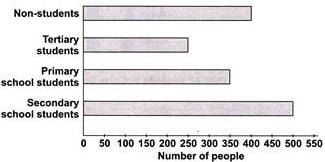Q. How many less tertiary students attended book fair than primary school students?

Detailed Solution for Test: Data Handling - 2 - Question 7

Number of primary school students = 350 Number of tertiary students = 250
∴ Difference = 350 − 250 =100 So, 100 less tertiary students visited book fair than primary school students.

Test: Data Handling - 2 - Question 8

DIRECTION: The given graph shows the number of people who attended a book fair. Study it carefully and answer the following questions.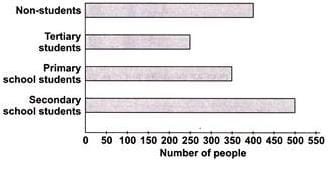Q. Number of secondary school students are ______ more than non-students.

Detailed Solution for Test: Data Handling - 2 - Question 8

Number of secondary school students = 500 Number of non-students = 400
∴ Difference = 500 − 400 =100 So, 100 more secondary school students visited book fair than non-students.

Test: Data Handling - 2 - Question 9

DIRECTION: The given pictograph shows the number of 4 different kinds of plants Mohit bought. Study the pictograph and answer the questions.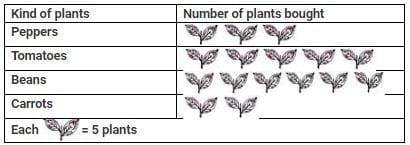Q. How many more tomato plants did he buy than pepper plants?

Detailed Solution for Test: Data Handling - 2 - Question 9

Number of tomato plants he bought = 5 × 5 = 25
Number of pepper plants he bought = 3 × 5 = 15
∴ Difference = 25 − 15 =10 So, he bought 10 more tomato plants.

Test: Data Handling - 2 - Question 10

DIRECTION: The given pictograph shows the number of 4 different kinds of plants Mohit bought. Study the pictograph and answer the questions.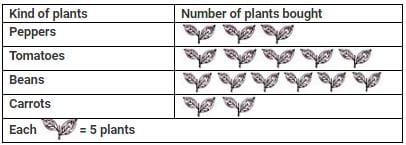Q. How many bean plants did he buy?

Detailed Solution for Test: Data Handling - 2 - Question 10

Number of bean plants he bought = 6 × 5 = 30

## Mathematics for Class 3: NCERT

51 videos|84 docs|44 tests
 Use Code STAYHOME200 and get INR 200 additional OFF Use Coupon Code
Information about Test: Data Handling - 2 Page
In this test you can find the Exam questions for Test: Data Handling - 2 solved & explained in the simplest way possible. Besides giving Questions and answers for Test: Data Handling - 2, EduRev gives you an ample number of Online tests for practice

## Mathematics for Class 3: NCERT

51 videos|84 docs|44 tests